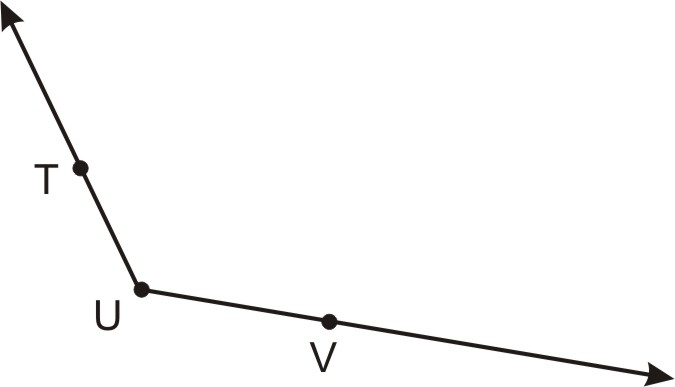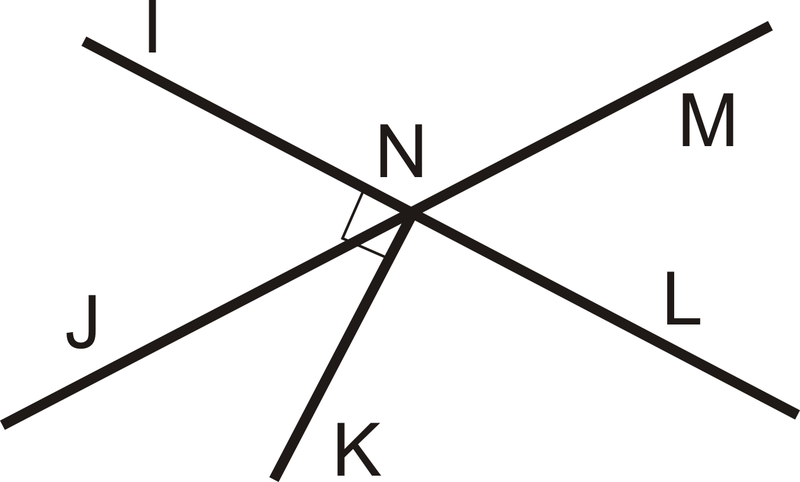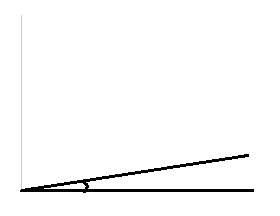Acute, Obtuse, Straight, or Right?
Name That Vertex!
Degrees of an Angle
Angle Pairs
Classifying Triangles
100

Classify the following angle.Acute

100

Name the vertex on the angle. (Vertex is where they meet)B

100

What is an angle called that measures 180 degrees?

Straight

100

Vertical angles are always __________

Congruent

100

What is a triangle with one 90 degree angle called?

Right triangle (Show someone your drawing.)

200

Classify the following angle.Straight

200

Name the vertex on the angle.U

200

What is an angle called that is less than 90 degrees?

Acute

200

What is the sum of two supplementary angles?

180 degrees

200

What is a triangle with one angle greater than 90 degrees called?

Obtuse triangle (Show someone your drawing.)

300

Classify the following angle.Right

300

Name the vertex on the angle.E

300

What is the name of an angle that measures 90 degrees?

Right

300

What are angles that are next to each other called?

300

What is a 90 degree angle called? Draw it.

Complimentary angle. (Show someone your drawing.)

400

Classify the following angle.Obtuse

400

Name the vertex on the angle.N

400

Acute, obtuse, right or straight? 173o

Obtuse

400

Two angles that make a line are called a ____?

Linear pair

400

This triangle has the following angles, 85 degrees, 65 degrees and 30 degrees.  What type of triangle is this?

Acute triangle

500

Classify the following angle.Acute

500

Name the vertex on the angle.Q

500

Acute, obtuse, right or straight? 15o

Acute

500

What is the sum of two complementary angles?

90 degrees

500

This triangle has at least three equal (60 degree) angles.  What type of triangle is this?

Equilateral triangle

Click to zoom